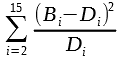# CHITEST gives unexpected value

I have two columns denoting respectively observed and expected frequency, I then calculate (chi-square) for each row with `((OBSERVED-EXPECTED)^2)/EXPECTED)` and proceed to `SUM` all rows to get the actual value. The resulting value is different from the value given by `CHITEST` and I can’t seem to understand why (in fact, I can’t really find much documentation about this function at all).
Also, the values in my table are rounded for display.

``````Observed    Expected  X²
3           4.0       0.26
15          18.5      0.66
47          42.5      0.47
76          65.2      1.78
68          75.0      0.66
74          69.0      0.36
46          52.9      0.90
39          34.8      0.52
15          20.0      1.25
9           10.2      0.15
5           4.7       0.02
2           2.0       0.00
0           0.8       0.75
1           0.3       2.02

SUM: 9.782  CHITEST: 0.712
``````

Could someone explain to me how is the value of `CHITEST` calculated?
The function CHITEST is implemented in ScInterpreter::ScChiTest. In your spreadsheet, given the `χ²` (result of) in E17, the function is equal to
``````=CHIDIST(E17;13)